## Practice Questions – Misc Aptitude – 1

1. The owner of a gift shop charges his customer 44 % more than the cost price. If a customer paid Rs. 648 for on wallet, then what was the cost price of the wallet?

(a) Rs. 575

(b) Rs. 435

(c) Rs. 450

(d) Rs. 515

(e) None of these

2. In an examination it is required to get 210 of the aggregate marks to pass. A student gets 168 marks and is declared failed by 8 % marks. What are the maximum aggregate marks a student can get?

(a) 635

(b) 575

(c) 445

(d) Cannot be determined

(e) None of these

3. The average age of a woman and her daughter is 21 yr. The ratio of their ages is 5 : 1 respectively. What will be ratio of their ages after 5 yr?

(a) 10 :3

(b) 5 : 2

(c) 4 :1

(d) 3 : 1

(e) None of these

4. Find the average of the following set of scores

345, 231, 777, 560, 892, 443, 546

(a) 545

(b) 540

(c) 542

(d) 548

(e) None of these

5. The cost of 4 Bowls and 6 Bottles is Rs. 142.5. What is the cost of 8 Bowls and 12 bottles?

(a) Rs. 445

(b) Rs. 285

(c) Rs. 345

(d) Cannot be determined

(e) None of these

6. 63 % of a number is 2583. What is 45 % of that number?

(a) 1845

(b) 1763

(c) 2255

(d) 1927

(e) None of these

7. If the fractions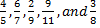are arranged in descending order of their values, which one will be the fourth?

(a) 9/11

(b) 4/5

(c) 3/8

(d) 6/7

(e) None of these

8. In an annual examination Haardik scores a total of 500 marks out of 725. What is his approximate percentage in the annual examination?

(a) 88

(b) 79

(c) 54

(d) 62

(e) 70

9. A canteen required 45 kg of rice for 3 days. Totally how many kg of rice will it required for the months of June, July and August 2008?

(a) 1380 kg

(b) 1365 kg

(c) 1395 kg

(d) 1410 kg

(e) None of these

10. 30 % of first number is 75 % of the second number. What is the respective ratio of the first number to the second number?

(a) 5  : 2

(b) 5 : 3

(c) 25 : 14

(d) Cannot be determined

(e) None of these

1. (c) 2. (e) 3. (a) 4. (c) 5. (b) 6. (a) 7. (c) 8. (e) 9. (a) 10. (a)

### Explanations

1. Cost price of the wallet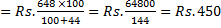2. Let the maximum aggregate marks be x.

Therefore, 8 % x = 210 – 168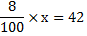X= 525

3. Let the ages of women and her daughter are 5x and x respectively.

Therefore,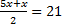6x = 42

X = 7

After 5 yr,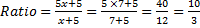4. The average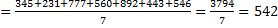5. Cost of 4 Bowls and 6 Bottles = Rs. 142.5

Cost of 8 Bowls and 12 bottles = 2×142.5 = Rs.285

6. Let the number be x.

63 % of x = 2583

1 % of x = 2583/63

45 % of x = 2583/63 × 45 = 1845

7.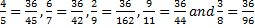In descending order,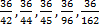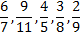8. His percentage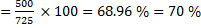9. Days in June, July and august 2008 = (30 + 31 + 31) days = 92 days

A canteen requires for 3 days = 45 kg

A canteen requires for 92 days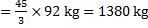10. Let the first and second numbers are x and y, respectively.

Therefore,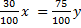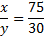x : y = 5 : 2